# Spiky strings lightlike Wilson loops and a ppwave

• Slides: 33
Download presentation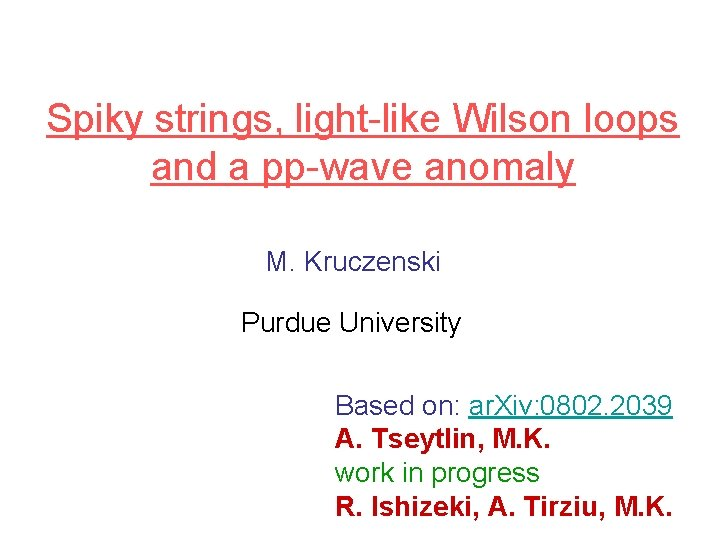Spiky strings, light-like Wilson loops and a pp-wave anomaly M. Kruczenski Purdue University Based on: ar. Xiv: 0802. 2039 A. Tseytlin, M. K. work in progress R. Ishizeki, A. Tirziu, M. K.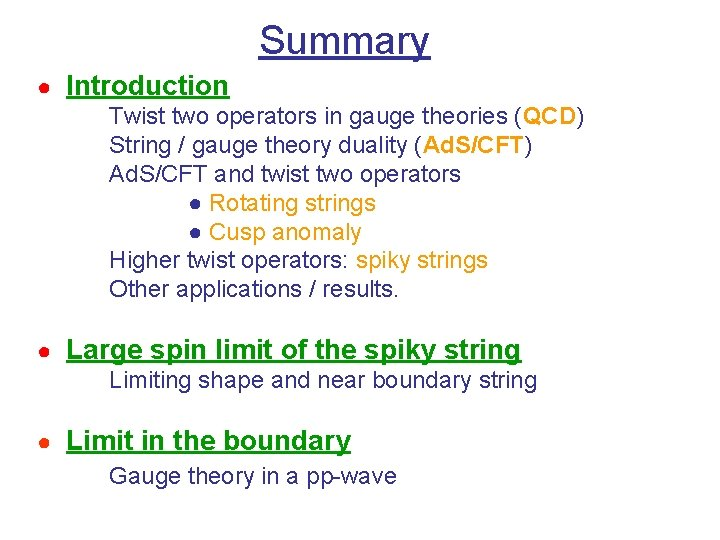Summary ● Introduction Twist two operators in gauge theories (QCD) String / gauge theory duality (Ad. S/CFT) Ad. S/CFT and twist two operators ● Rotating strings ● Cusp anomaly Higher twist operators: spiky strings Other applications / results. ● Large spin limit of the spiky string Limiting shape and near boundary string ● Limit in the boundary Gauge theory in a pp-wave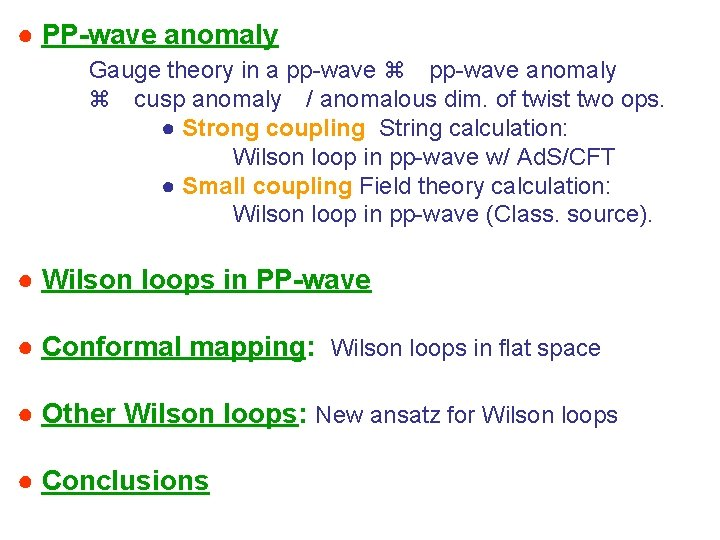● PP-wave anomaly Gauge theory in a pp-wave anomaly cusp anomaly / anomalous dim. of twist two ops. ● Strong coupling String calculation: Wilson loop in pp-wave w/ Ad. S/CFT ● Small coupling Field theory calculation: Wilson loop in pp-wave (Class. source). ● Wilson loops in PP-wave ● Conformal mapping: Wilson loops in flat space ● Other Wilson loops: New ansatz for Wilson loops ● ConclusionsTwist two operators in gauge theories (QCD) e e q 2 ∞, =-2 p. q / q 2 fixed g, q H, p X or q+ ∞, q- fixed Near l. c. expansion OPE: (z 2 0, light-like, twist), (z 2 0, euclidean, conf. dim. This (after including indices correctly) is plugged into:String/gauge theory duality: Large N limit (‘t Hooft) QCD [ SU(3) ] q Large N-limit [SU(N)] q Effective strings Strong coupling More precisely: (‘t Hooft coupl. ) Lowest order: sum of planar diagrams (infinite number)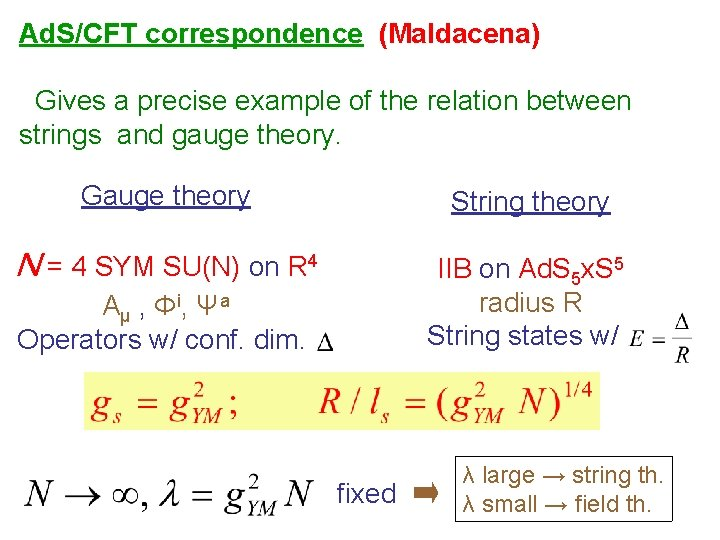Ad. S/CFT correspondence (Maldacena) Gives a precise example of the relation between strings and gauge theory. Gauge theory String theory N = 4 SYM SU(N) on R 4 IIB on Ad. S 5 x. S 5 radius R String states w/ Aμ , Φi, Ψa Operators w/ conf. dim. fixed λ large → string th. λ small → field th.Twist two operators from rotation in Ad. S 5 (Gubser, Klebanov, Polyakov) θ = ω tTwist two ops. from cusp anomaly (MK, Makeenko) The anomalous dimensions of twist two operators can also be computed by using the cusp anomaly of light-like Wilson loops (Korchemsky and Marchesini). In Ad. S/CFT Wilson loops can be computed using surfaces of minimal area in Ad. S 5 (Maldacena, Rey, Yee) z The result agrees with the rotating string calculation.Generalization to higher twist operators (MK) In flat space such solutions are easily found in conf. gaugSpiky strings in Ad. S: Ansatz: Action and momenta: Eq. of motion:For all couplings we are lead to define f(l) through: E = S + (n/2) f(l) ln S (large S) Other applications / results for f(l) ● Gluon scattering amplitudes (Bern, Dixon, Smirnov …) ● Scattering amplitudes in Ad. S/CFT (Alday, Maldacena, …) ● Anomalous dimension f(l) at all loops (Beisert, Eden, Staudacher , …)Large spin limit of the spiky string The limit of large spin (E-S ~ ln S) corresponds to 1. In that limit the spikes touch the boundary and the solution simplifies. In embedding coordinates The same f(l) should appear in all of them!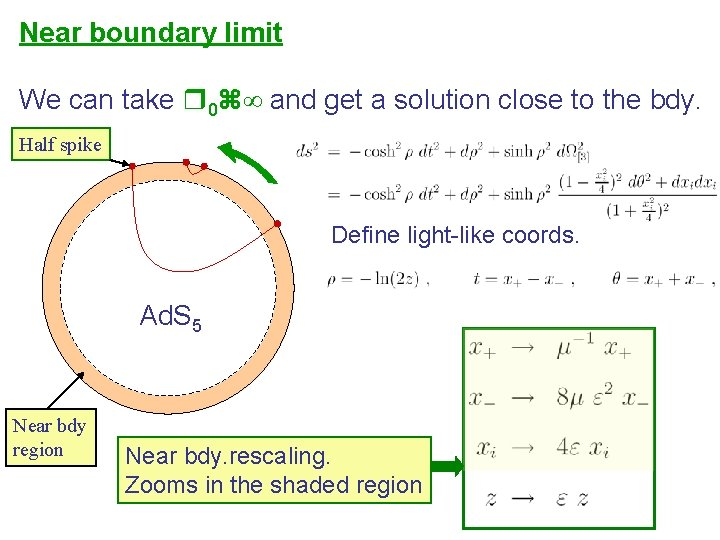Near boundary limit We can take 0 ∞ and get a solution close to the bdy. Half spike Define light-like coords. Ad. S 5 Near bdy region Near bdy. rescaling. Zooms in the shaded regionIn the limit 0 we get the metric: Ad. S! So, the tiny string sees an Ad. S pp-wave in Poincare coordinates. When z 0, the boundary metric becomes a pp-wave in usual flat space: N = 4 SYM SU(N) on R 4 pp-wave dual to IIB on Ad. S 5 pp-wave x S 5 According to Ad. S/CFT This duality should contain all the information about f(l)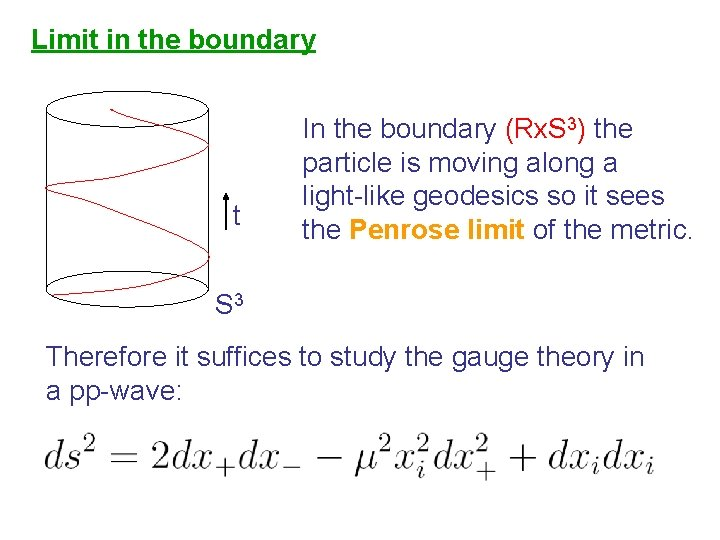Limit in the boundary t In the boundary (Rx. S 3) the particle is moving along a light-like geodesics so it sees the Penrose limit of the metric. S 3 Therefore it suffices to study the gauge theory in a pp-wave: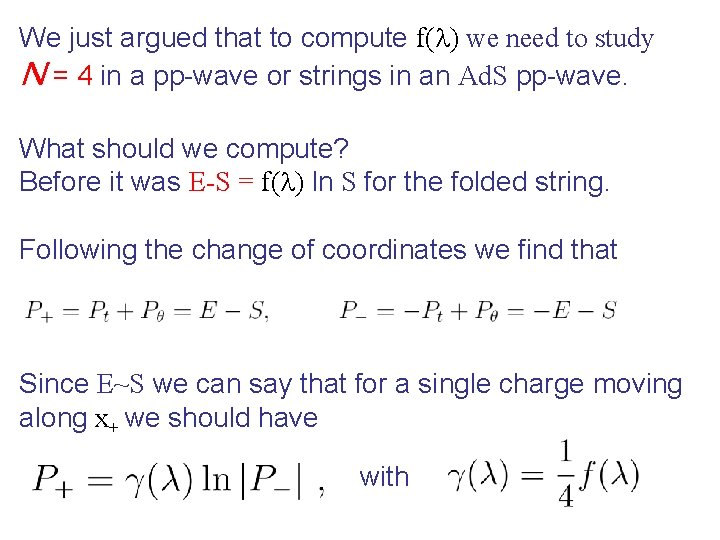We just argued that to compute f(l) we need to study N = 4 in a pp-wave or strings in an Ad. S pp-wave. What should we compute? Before it was E-S = f(l) ln S for the folded string. Following the change of coordinates we find that Since E~S we can say that for a single charge moving along x+ we should have with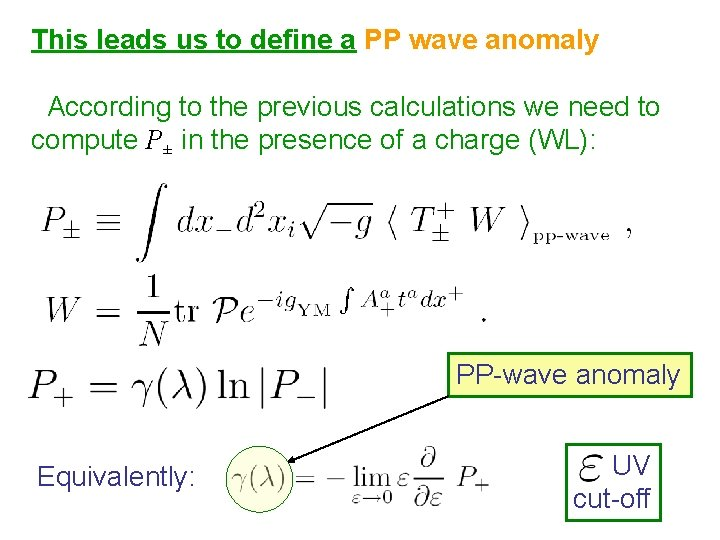This leads us to define a PP wave anomaly According to the previous calculations we need to compute P± in the presence of a charge (WL): PP-wave anomaly Equivalently: UV cut-offPP wave anomaly Strong coupling We put a particle in a pp-wave background and compute P± which amounts to computing a Wilson loop. We can use Ad. S/CFT since we know That the dual of the pp-wave is the Ad. S pp-wave. The Wilson loop is x+=t and the string solution is simply: Giving: or OK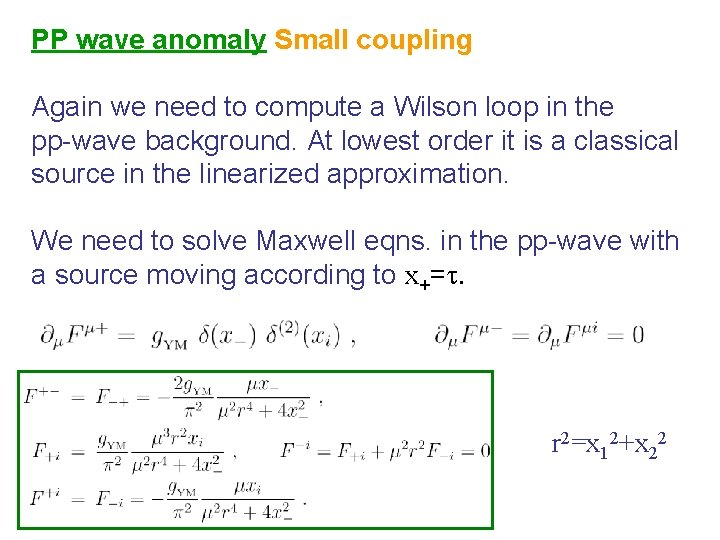PP wave anomaly Small coupling Again we need to compute a Wilson loop in the pp-wave background. At lowest order it is a classical source in the linearized approximation. We need to solve Maxwell eqns. in the pp-wave with a source moving according to x+=t. r 2=x 12+x 22We can now compute the energy momentum tensor Obtaining Using we get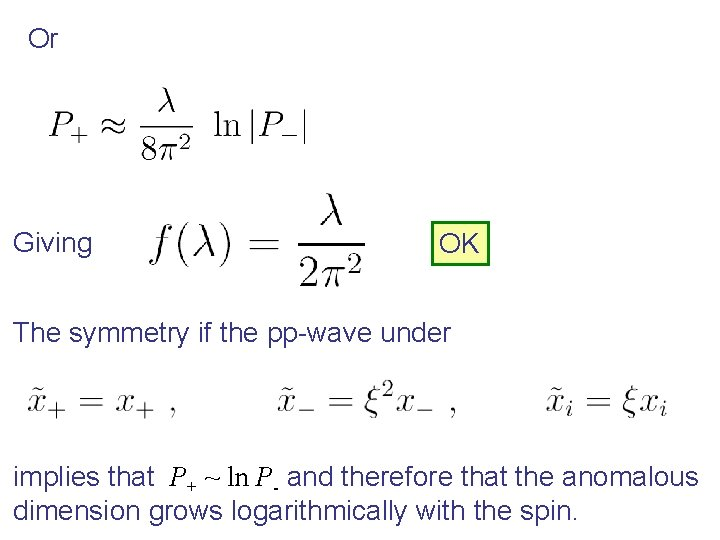Or Giving OK The symmetry if the pp-wave under implies that P+ ~ ln P- and therefore that the anomalous dimension grows logarithmically with the spin.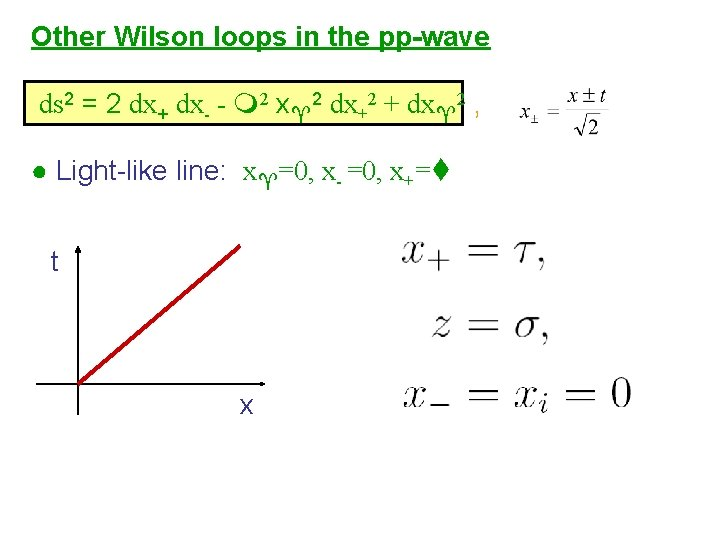Other Wilson loops in the pp-wave ds 2 = 2 dx+ dx- - 2 x 2 dx+2 + dx 2 , ● Light-like line: x =0, x- =0, x+= t x● Time-like line: x= x =0, t= t x ● Parallel lines in x+ direction: x =0 , x- =±a, x+= t x● Parallel lines in x+ direction: x 2=a, b , x- =0, x+= t x x 2 ● Parallel line in time-like direction: x=±a, t= t xConformal mapping The metric: ds 2 = 2 dx+ dx- - 2 x 2 dx+2 + dx 2 , is conformally flat! But this is a local equivalence. Equivalently the Ad. S pp-wave is (locally) Ad. S space in different coordinates. (Brecher, Chamblin, Reall) We can map the Wilson loop solutions to Wilson loops in ordinary space.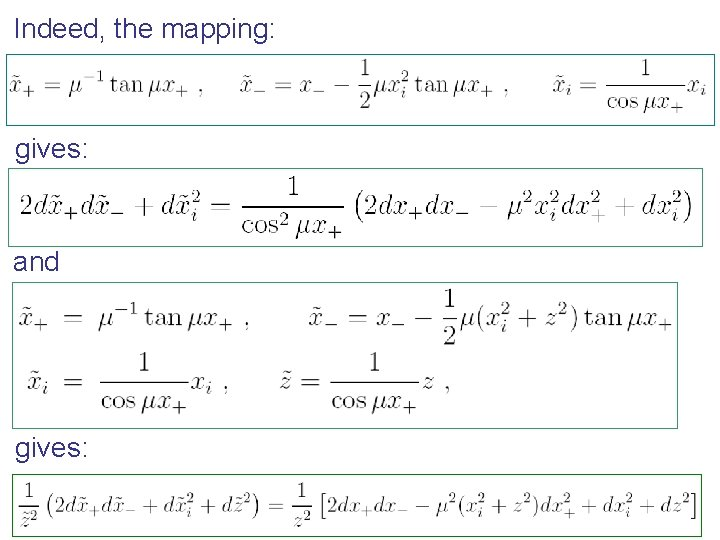Indeed, the mapping: gives: and gives: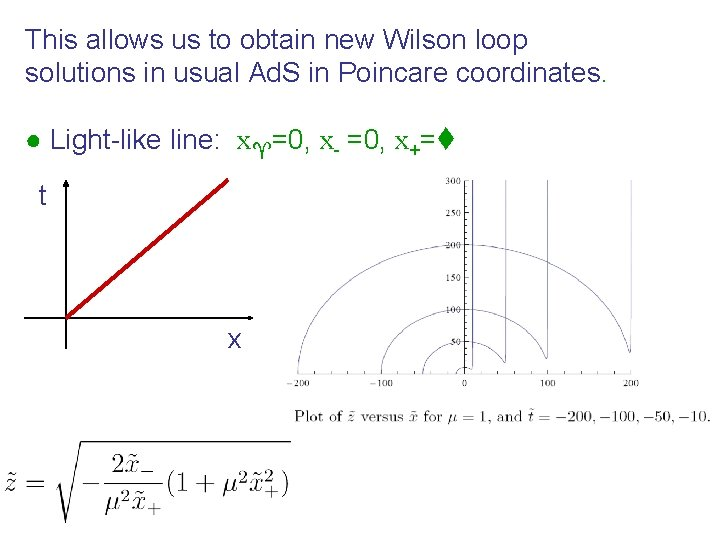This allows us to obtain new Wilson loop solutions in usual Ad. S in Poincare coordinates. ● Light-like line: x =0, x- =0, x+= t x● Time-like line: x= x =0, t= The Wilson loop now changes shape. Particle decelerating and then accelerating. t x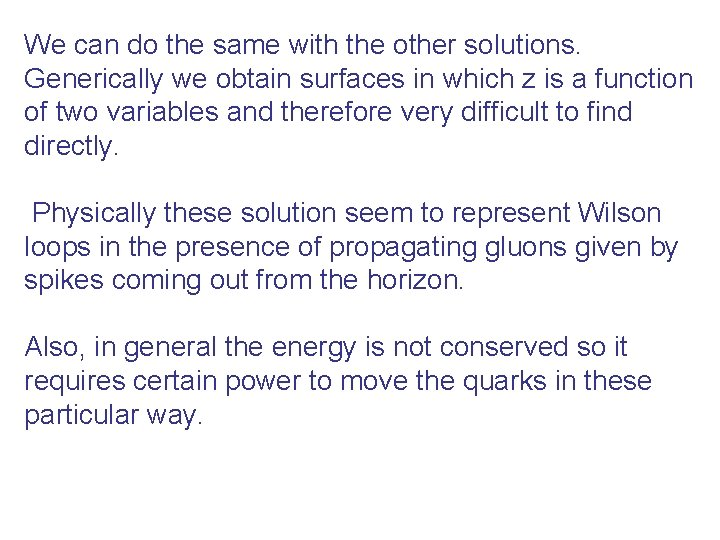We can do the same with the other solutions. Generically we obtain surfaces in which z is a function of two variables and therefore very difficult to find directly. Physically these solution seem to represent Wilson loops in the presence of propagating gluons given by spikes coming out from the horizon. Also, in general the energy is not conserved so it requires certain power to move the quarks in these particular way.Other Wilson loop solutions (new ansatz) We use the ansatz The equations of motion linearize. We get: The boundary curve (WL) is: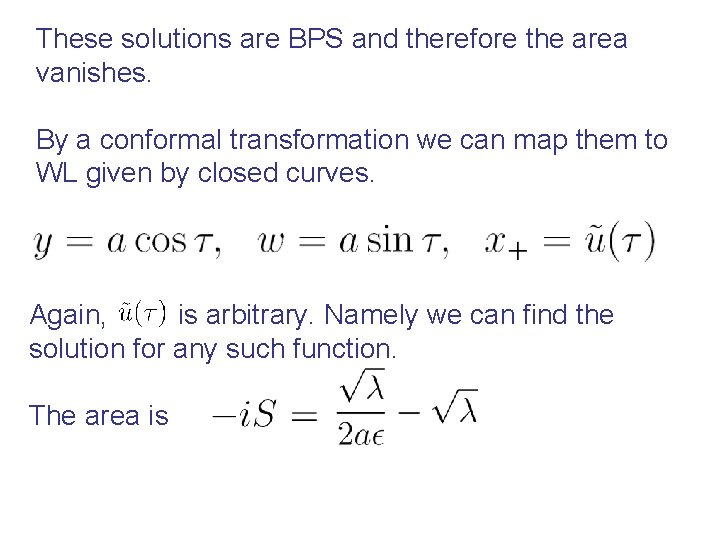These solutions are BPS and therefore the area vanishes. By a conformal transformation we can map them to WL given by closed curves. Again, is arbitrary. Namely we can find the solution for any such function. The area is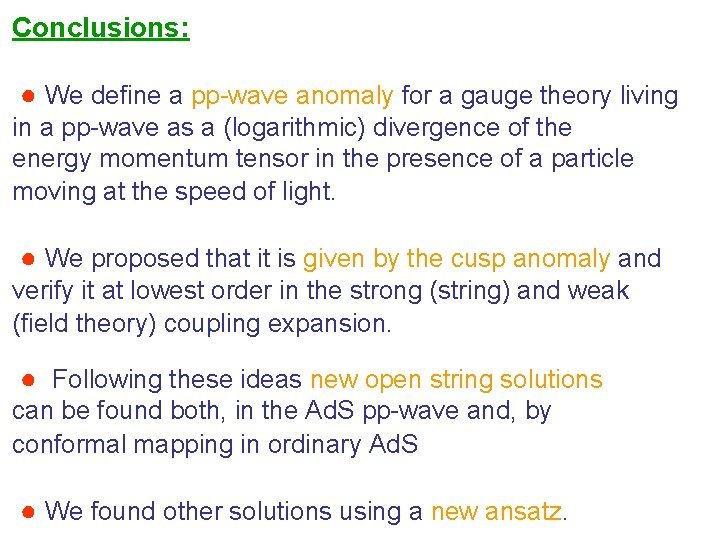Conclusions: ● We define a pp-wave anomaly for a gauge theory living in a pp-wave as a (logarithmic) divergence of the energy momentum tensor in the presence of a particle moving at the speed of light. ● We proposed that it is given by the cusp anomaly and verify it at lowest order in the strong (string) and weak (field theory) coupling expansion. ● Following these ideas new open string solutions can be found both, in the Ad. S pp-wave and, by conformal mapping in ordinary Ad. S ● We found other solutions using a new ansatz.